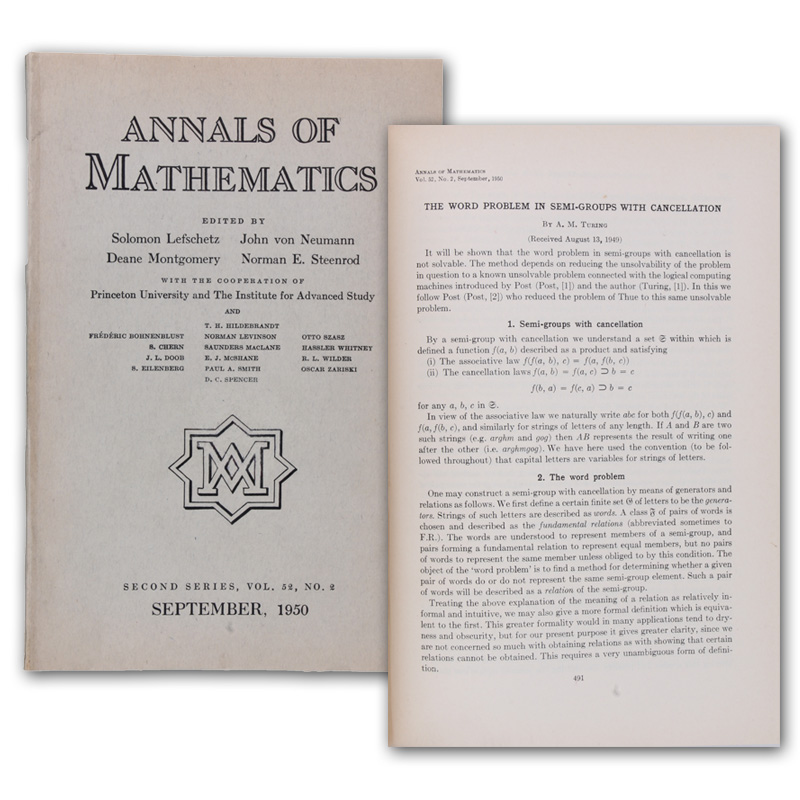# Artikel 111740-01111740-01  Turing, A(lan) M(athison).
The word problem in semi-groups with cancellation. SS. (491)-505. In: Annals of Mathematics. Vol. 52, No. 2. (24 x 16 cm). SS. (245)-507. Mit Abbildungen. Original-Broschur.
Princeton, University Press, 1950.
Erste Ausgabe dieser klassischen Arbeit der theoretischen Informatik. "In 1953 (richtig 1950) Turing wrote his last academic research paper in pure mathematics: 'The word problem in semi-groups with cancellation'. Given a set with an associative multiplication operation (a semi-group), obeying additionally the cancellation laws ab=ac (imply) b=c and ba=ca (imply) b=c, can there be such, which has a presentation in terms of two finite sets of symbols and of equations, but so that the problem of deciding whether two 'words'... each made up of a string of symbolmultiplicands are in fact equal, is unsolvable? In 1947 such a problem had been shown unsolvable by Post and Markov independently for pure semi-groups. In this paper Turing shows that here too the general problem is unsolvable. As (Boone) states, this argument was to be the basis of both Boone's and Novikov's (independent) proofs of the unsolvability of the word problem for groups" (Welch, Turing's Mathematical Work S. 14). - Rücken sauber erneuert, sonst wohlerhalten. - DSB 13, 497